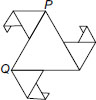# User ForumSubject :IMO    Class : Class 7

In the given figure, all triangles are equilateral and PQ = 12 units. Other triangles have been formed by taking the mid points of the sides. What is the perimeter of the figure?A 62.3 units
B 64.5 units
C 65.8 units
D 67.5 units

can anyone explain this

Class : Class 8

## Ans 2: (Master Answer)

Class : Class 1

The answer is D

Perimeter of the given figure
= 9 × 1.5 + 6 × 3 + 6 × 6
= (13.5 + 18 + 36) units = 67.5 units

Class : Class 7

## Ans 4:

Class : Class 7
thank you very much